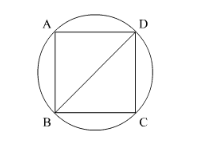# The area of a circle is 220 cm2.Question:

The area of a circle is $220 \mathrm{~cm}^{2}$. The area of ta square inscribed in it is

(a) $49 \mathrm{~cm}^{2}$

(b) $70 \mathrm{~cm}^{2}$

(c) $140 \mathrm{~cm}^{2}$

(d) $150 \mathrm{~cm}^{2}$

Solution:Let BD be the diameter and diagonal of the circle and the square respectively.

We know that area of the circle $=\pi r^{2}$

Area of the circle $=\pi r^{2}$

$\therefore 220=\frac{22}{7} \times r^{2}$

Multiplying both sides of the equation by 7 we get,

$220 \times 7=22 \times r^{2}$

Dividing both sides of the equation by 22 we get,

$\therefore r^{2}=70$

$\therefore r=\sqrt{70}$

As we know that diagonal of the square is the diameter of the square.

$\therefore$ Diagonal $=2 r$

$\therefore$ Diagonal $=2 \sqrt{70}$

$\therefore$ Side of the square $=\frac{\text { diagonal }}{\sqrt{2}}$.......(1)

Substituting Diagonal $=2 \sqrt{70}$ in equation (1) we get,

Side of the square $=\frac{2 \sqrt{70}}{\sqrt{2}}$

$\therefore$ Side of the square $=2 \sqrt{\frac{70}{2}}$

$\therefore$ Side of the square $=2 \sqrt{35}$

$\therefore$ Area of the square $=\operatorname{side}^{2}$

$=(2 \sqrt{35})^{2}$

$=4 \times 35$

$=140$

Therefore, area of the square is $140 \mathrm{~cm}^{2}$

Hence, the correct answer is option (c).